##### The Japanese Bonsai specialist
Direct order Contact Help / Services Newsletter# Bonsai scissor 180 mm

› Bonsai tools › Training tools › Pruning scissors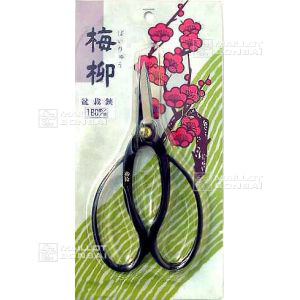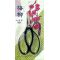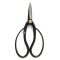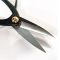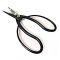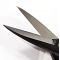ref. : 4441

29,00

Available quantity : 5Order

###### Description

This "okubo asami" design of scissor is one of the original tools used in early bonsai culture. These are Japanese manufacture and are for trimming branches and roots. Good quality steel, unlike similar scissors made in China. Easy sharpening with a whetstone. Length 180 millimetres.

#scissors 3.8 #scissor 3.7 #bonsai 3.6 #pruning 2.7 #manufacture 2.1 #millimetres 2.1 #sharpening 2 #whetstone 1.9 #original 1.8 #branches 1.8

Formule
(( ROUND((CHAR_LENGTH(b.article_nom)-CHAR_LENGTH(REPLACE(b.article_nom, 'scissor', '')))/LENGTH('scissor')) + ROUND((CHAR_LENGTH(b.article_description)-CHAR_LENGTH(REPLACE(b.article_description, 'scissor', '')))/LENGTH('scissor')) ) * 3.7) + (( ROUND((CHAR_LENGTH(b.article_nom)-CHAR_LENGTH(REPLACE(b.article_nom, 'bonsai', '')))/LENGTH('bonsai')) + ROUND((CHAR_LENGTH(b.article_description)-CHAR_LENGTH(REPLACE(b.article_description, 'bonsai', '')))/LENGTH('bonsai')) ) * 3.6) + (( ROUND((CHAR_LENGTH(b.article_nom)-CHAR_LENGTH(REPLACE(b.article_nom, 'millimetres', '')))/LENGTH('millimetres')) + ROUND((CHAR_LENGTH(b.article_description)-CHAR_LENGTH(REPLACE(b.article_description, 'millimetres', '')))/LENGTH('millimetres')) ) * 2.1) + (( ROUND((CHAR_LENGTH(b.article_nom)-CHAR_LENGTH(REPLACE(b.article_nom, 'manufacture', '')))/LENGTH('manufacture')) + ROUND((CHAR_LENGTH(b.article_description)-CHAR_LENGTH(REPLACE(b.article_description, 'manufacture', '')))/LENGTH('manufacture')) ) * 2.1) + (( ROUND((CHAR_LENGTH(b.article_nom)-CHAR_LENGTH(REPLACE(b.article_nom, 'sharpening', '')))/LENGTH('sharpening')) + ROUND((CHAR_LENGTH(b.article_description)-CHAR_LENGTH(REPLACE(b.article_description, 'sharpening', '')))/LENGTH('sharpening')) ) * 2) + (( ROUND((CHAR_LENGTH(b.article_nom)-CHAR_LENGTH(REPLACE(b.article_nom, 'whetstone', '')))/LENGTH('whetstone')) + ROUND((CHAR_LENGTH(b.article_description)-CHAR_LENGTH(REPLACE(b.article_description, 'whetstone', '')))/LENGTH('whetstone')) ) * 1.9) + (( ROUND((CHAR_LENGTH(b.article_nom)-CHAR_LENGTH(REPLACE(b.article_nom, 'original', '')))/LENGTH('original')) + ROUND((CHAR_LENGTH(b.article_description)-CHAR_LENGTH(REPLACE(b.article_description, 'original', '')))/LENGTH('original')) ) * 1.8) + (( ROUND((CHAR_LENGTH(b.article_nom)-CHAR_LENGTH(REPLACE(b.article_nom, 'japanese', '')))/LENGTH('japanese')) + ROUND((CHAR_LENGTH(b.article_description)-CHAR_LENGTH(REPLACE(b.article_description, 'japanese', '')))/LENGTH('japanese')) ) * 1.8) + (( ROUND((CHAR_LENGTH(b.article_nom)-CHAR_LENGTH(REPLACE(b.article_nom, 'scissors', '')))/LENGTH('scissors')) + ROUND((CHAR_LENGTH(b.article_description)-CHAR_LENGTH(REPLACE(b.article_description, 'scissors', '')))/LENGTH('scissors')) ) * 1.8) + (( ROUND((CHAR_LENGTH(b.article_nom)-CHAR_LENGTH(REPLACE(b.article_nom, 'trimming', '')))/LENGTH('trimming')) + ROUND((CHAR_LENGTH(b.article_description)-CHAR_LENGTH(REPLACE(b.article_description, 'trimming', '')))/LENGTH('trimming')) ) * 1.8)

## Secure payment## Delivery

Our logistic partners :04 74 55 23 48
Pépinière MAILLOT-BONSAÏ
Le Bois Frazy
01990 RELEVANT - FRANCE
on appointment